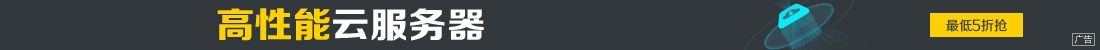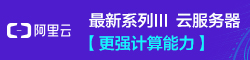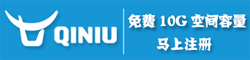# JavaScript中的ubound函数使用实例

••JavaScript中ubound函数方法是返回在 VBArray 的指定维中所使用的最大索引值。使用方法：

safeArray.ubound(dimension)

dimension是可选项。要获知其索引上界的 VBArray 的维数。如果忽略，ubound 将该参数作为 1 进行处理。

<SCRIPT LANGUAGE="VBScript">
<!--
Function CreateVBArray()
Dim i, j, k
Dim a(2, 2)
k = 1
For i = 0 To 2
For j = 0 To 2
a(j, i) = k
k = k + 1
Next
Next
CreateVBArray = a
End Function
-->
</SCRIPT>

<SCRIPT LANGUAGE="JScript">
<!--
function VBArrayTest(vba)
{
var i, s;
var a = new VBArray(vba);
for (i = 1; i <= a.dimensions(); i++)
{
s = "The upper bound of dimension ";
s += i + " is ";
s += a.ubound(i)+ ".
";
return(s);
}
}
-->
</SCRIPT>

<BODY>
<SCRIPT language="jscript">
document.write(VBArrayTest(CreateVBArray()));
</SCRIPT>
</BODY>